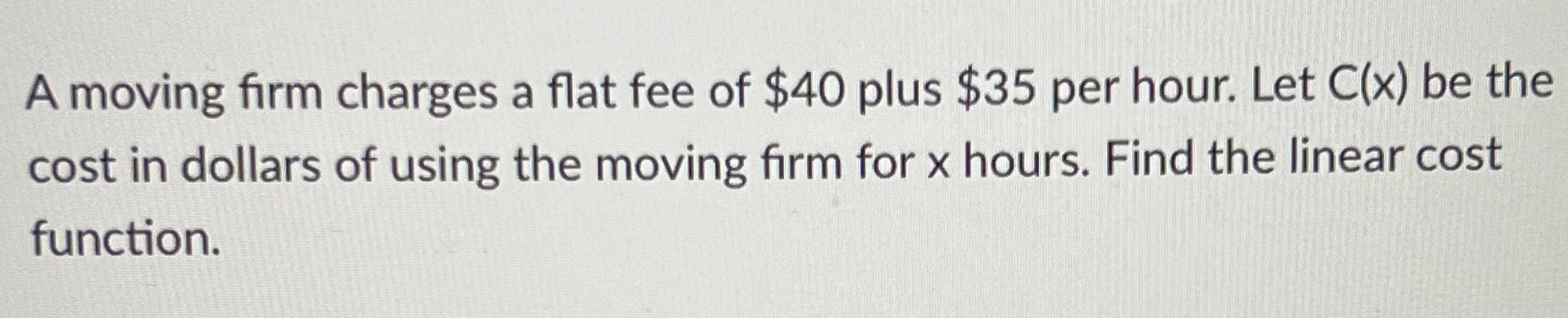### ¿Todavía tienes preguntas de matemáticas?

Pregunte a nuestros tutores expertos
Algebra
PreguntaA moving firm charges a flat fee of $$\ 40$$ plus $$\ 35$$ per hour. Let $$C ( x )$$ be the cost in dollars of using the moving firm for $$x$$ hours. Find the linear cost function.